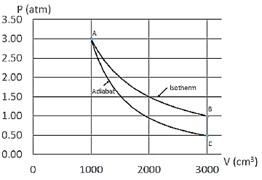# Problem: An ideal gas is taken through the cycle shown in the diagram below. The process is carried out with 150 mg of Helium, which is a monoatomic gas with an atomic mass of 4 g/mol. First, the gas undergoes an adiabatic expansion (from point A to point C), followed by an increase in pressure at constant volume (from point C to point B). Finally, the gas is taken back to the initial state by an isothermal compression (from point B to point A). What is the total work done on the system? Note that the work during an isothermal process is W = nRT ln(V i / Vf).

###### FREE Expert Solution
99% (319 ratings)
###### Problem Details

An ideal gas is taken through the cycle shown in the diagram below. The process is carried out with 150 mg of Helium, which is a monoatomic gas with an atomic mass of 4 g/mol. First, the gas undergoes an adiabatic expansion (from point A to point C), followed by an increase in pressure at constant volume (from point C to point B). Finally, the gas is taken back to the initial state by an isothermal compression (from point B to point A). What is the total work done on the system? Note that the work during an isothermal process is W = nRT ln(V i / Vf).Frequently Asked Questions

What scientific concept do you need to know in order to solve this problem?

Our tutors have indicated that to solve this problem you will need to apply the Work & PV Diagrams concept. You can view video lessons to learn Work & PV Diagrams. Or if you need more Work & PV Diagrams practice, you can also practice Work & PV Diagrams practice problems.

How long does this problem take to solve?

Our expert Physics tutor, Jeffery took 7 minutes and 59 seconds to solve this problem. You can follow their steps in the video explanation above.

What professor is this problem relevant for?

Based on our data, we think this problem is relevant for Professor Loftin's class at MIZZOU.